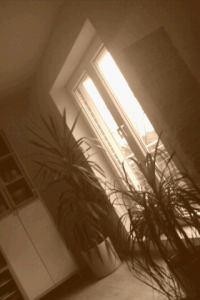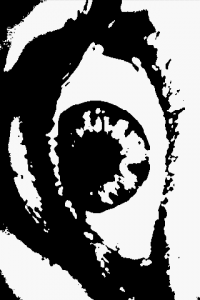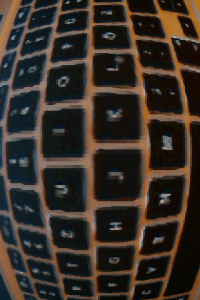# Camera image->NDK->OpenGL texture

Since we are currently working on some augmented reality stuff for Android I need to show the camera image using OpenGL ES. It works great with pure Java if one uses only the grayscale image. However, I needed the color image. The G1’s camera delivers the image in a YUV format while OpenGL only understand RGB images. Unfortunately it is out of question to convert the YUV image to RGB in pure Java for images with 480×320 pixels. Thus, I used the NDK to implement the conversion. The code below does the job. It is based on code provided by Tom Gibara.

```void toRGB565(unsigned short *yuvs, int widthIn, int heightIn, unsigned int *rgbs, int widthOut, int heightOut) {
int half_widthIn = widthIn >> 1;

//the end of the luminance data
int lumEnd = (widthIn * heightIn) >> 1;
//points to the next luminance value pair
int lumPtr = 0;
//points to the next chromiance value pair
int chrPtr = lumEnd;
//the end of the current luminance scanline
int lineEnd = half_widthIn;

int x,y;
for (y=0;y> 1;
for (x=0;x> 8) & 0xff;
Y1 = Y1 & 0xff;
int Cr = yuvs[chrPtr++];
int Cb = ((Cr >> 8) & 0xff) - 128;
Cr = (Cr & 0xff) - 128;

int R, G, B;
//generate first RGB components
B = Y1 + ((454 * Cb) >> 8);
if (B < 0) B = 0; if (B > 255) B = 255;
G = Y1 - ((88 * Cb + 183 * Cr) >> 8);
if (G < 0) G = 0; if (G > 255) G = 255;
R = Y1 + ((359 * Cr) >> 8);
if (R < 0) R = 0; if (R > 255) R = 255;
int val = ((R & 0xf8) << 8) | ((G & 0xfc) << 3) | (B >> 3);

//generate second RGB components
B = Y1 + ((454 * Cb) >> 8);
if (B < 0) B = 0; if (B > 255) B = 255;
G = Y1 - ((88 * Cb + 183 * Cr) >> 8);
if (G < 0) G = 0; if (G > 255) G = 255;
R = Y1 + ((359 * Cr) >> 8);
if (R < 0) R = 0; if (R > 255) R = 255;
rgbs[yPosOut+x] = val | ((((R & 0xf8) << 8) | ((G & 0xfc) << 3) | (B >> 3)) << 16);
}
//skip back to the start of the chromiance values when necessary
chrPtr = lumEnd + ((lumPtr  >> 1) / half_widthIn) * half_widthIn;
lineEnd += half_widthIn;
}
}
```

The code is not that optimized at the moment but can process a 480×320 image in ~25ms on my G1 (which is somewhat slow according to my student’s comments). In order to call this function from Java I needed a wrapper with a JNI signature:

```/**
* Converts the input image from YUV to a RGB 5_6_5 image.
* The size of the output buffer must be at least the size of the input image.
*/
JNIEXPORT void JNICALL Java_de_offis_magic_core_NativeWrapper_image2TextureColor
(JNIEnv *env, jclass clazz,
jbyteArray imageIn, jint widthIn, jint heightIn,
jobject imageOut, jint widthOut, jint heightOut,
jint filter) {

jbyte *cImageIn = (*env)->GetByteArrayElements(env, imageIn, NULL);

toRGB565((unsigned short*)cImageIn, widthIn, heightIn, (unsigned int*)cImageOut, widthOut, heightOut);

(*env)->ReleaseByteArrayElements(env, imageIn, cImageIn, JNI_ABORT);
}
```

To make it more interesting I added some filter to the camera image. There is a demo app in the market (direct link to the market). I tried to make the whole thing portable but would love to know if it works on other devices like the Motorola Milestone.# Processing camera frames on Android

Recently I wanted to process and display camera frames using my Android G1. I’ve done similar things using Python on S60 and Windows Mobile 6 and expected it to be quite easy on the G1 as well. As first step I extended a SurfaceView that uses the camera and calls setPreviewCallback to register a onPreviewFrame callback and receive images from the camera as described in several tutorials. The camera frames are then displayed via my SurfaceView and I receive the according data as well.

However, I wanted to keep the processing of the frames and displaying the frames in sync. With the simple approach this is not possible because the onPreviewFrame is not synchronized with displaying the frames. My alternative was to not display the frames with the SurfaceView directly but convert the received image data to an OpenGL texture and render the camera viewfinder with OpenGL ES. This works surprisingly fast on my G1. In the video below I render the camera frames on an OpenGL rectangle to get some fancy effects.

My viewfinder is grayscale because I only copy the luminance part of the camera frames (which is encoded in a YUV colour space) to the OpenGL texture. Decomposing also the U and V part is probably a bit slower. Copying a 160×240 YUV frame to a 256×256 luminance array (which is used to create the texture) is very simple and looks as follows.
``` public static void yuvToLum160x240(byte[] yuv, byte[] lum) { int lumCount=0; int yuvCount=0; for (int y=0;y<160;y++) { System.arraycopy(yuv, yuvCount, lum, lumCount, 240); yuvCount=yuvCount+240; lumCount=lumCount+256; } } ```